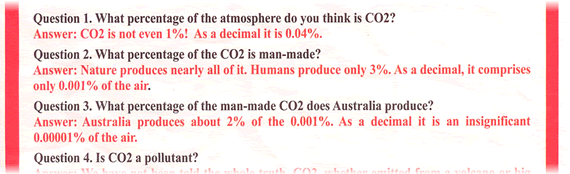# MathsClass

A blog about teaching and learning in a maths classroom.

## Carbon Tax Numbers

Monday, 11 July 2011 | Comments

I got this flyer in the mailbox today (extract)...The answer to the first question “CO2 is not even 1%! As a decimal it is 0.04%” immediately got me wondering, why say “as a decimal?” It’s just poor use of terminology, but I had a look at the rest of the numbers.

My purpose here is not to question the data, although Answer 1 seems correct. Wikipedia agrees “[Carbon dioxide] is a gas at standard temperature and pressure and exists in Earth’s atmosphere in this state, as a trace gas at a concentration of 0.039% by volume.” So, CATA have rounded up.

3% of 0.04% = 0.0012%. CATA have rounded down to 0.001%.

2% of 0.001% = 0.00002%
(If the 0.0012% figure had been used, that would be 0.000024%)
CATA have it as 0.00001%.
There is an error here.

Ways you could use this in class.
1. Find the error shown above.
2. Has this organisation represented the facts/numbers well?
(Get the students to Answers the question in mass rather than percentages.)
Answer 1. The mass figure from Wikipedia, “The total mass of atmospheric carbon dioxide is 3.16×1015 kg (about 3,000 gigatonnes)”.
Answer 2. 3% of 3.16×1015 kg = 9.48×1013 kg
Answer 3. 2% of 9.48×1013 kg = 1.896×1012 kg
That is, 1 896 000 000 000 kg. Wouldn’t have looked as good on the flyer.

Posted in • In the news | Short URL: http://mths.co/2234

• Share:

There are no comments for this entry yet.

Commenting is not available in this channel entry.

New  Subscribe to the …

Simon Job — eleventh year of teaching maths in a public high school in Western Sydney, Australia.
MathsClass is about teaching and learning in a maths classroom. more→

## Elsewhere@simonjob
updates via@mathslinks